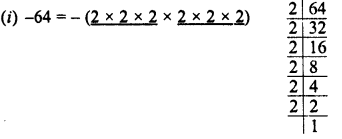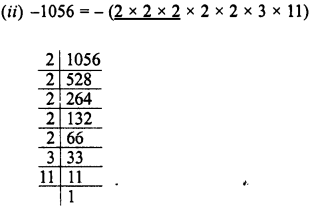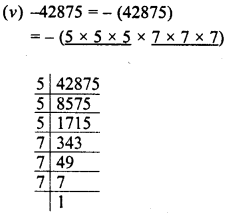# RD Sharma Class 8 Solutions Chapter 4 Cubes and Cube Roots Ex 4.2

In this chapter, we provide RD Sharma Class 8 Solutions Chapter 4 Cubes and Cube Roots Ex 4.2 for English medium students, Which will very helpful for every student in their exams. Students can download the latest RD Sharma Class 8 Solutions Chapter 4 Cubes and Cube Roots Ex 4.2 Maths pdf, free RD Sharma Class 8 Solutions Chapter 4 Cubes and Cube Roots Ex 4.2 Maths book pdf download. Now you will get step by step solution to each question.

### RD Sharma Solutions for Class 8 Chapter 4 Cubes and Cube Roots Ex 4.2Download PDF

Question 1.
Find the cubes of:
(i) -11
(ii) -12
(iii) – 21
Solution:
(i) (-11)3=(-11)3=(11 x 11 x 11) =-1331
(ii) (-12)3=(-12)3=(12 x 12 x 12) =  -1728
(iii) (-21)3=(-21)3=(21 x 21 x 21) = -9261

Question 2.
Which of the following numbers are cubes of negative integers.

(i) -64
(ii) -1056
(iii) -2197
(iv) -2744
(v)  -42875
Solution:∴ All factors of 64 can be grouped in triplets of the equal factors completely.
∴ -64 is a perfect cube of negative integer.All the factors of 1056 can be grouped in triplets of equal factors grouped completely
∴ 1058 is not a perfect cube of negative integer.All the factors of -2197 can be grouped in triplets of equal factors completely
∴ 2197 is a perfect cube of negative integer,All the factors of -2744 can be grouped in triplets of equal factors completely
∴ 2744 is a perfect cube of negative integerAll the factors of -42875 can be grouped in triplets of equal factors completely
∴ 42875 is a perfect cube of negative integer.

Question 3.
Show that the following integers are cubes of negative integers. Also, find the integer whose cube is the given integer :
(i) -5832 (ii) -2744000
Solution:Grouping the factors in triplets of equal factors, we see that no factor is left
∴ -5832 is a perfect cube
Now taking one factor from each triplet we find that
-5832 is a cube of – (2 x 3 x 3) = -18
∴ Cube root of-5832 = -18Grouping the factors in tuplets of equal factors, we see that no factor is left. Therefore it is a perfect cube.
Now taking one factor from each triplet, we find that.
-2744000 is a cube of – (2 x 2 x 5 x 7) ie. -140
∴ Cube root of -2744000 = -140

Question 4.
Find the cube of :Solution:Question 5.
Which of the following numbers are cubes of rational numbers :Solution:All Chapter RD Sharma Solutions For Class 8 Maths

—————————————————————————–

All Subject NCERT Exemplar Problems Solutions For Class 8

All Subject NCERT Solutions For Class 8

*************************************************

I think you got complete solutions for this chapter. If You have any queries regarding this chapter, please comment on the below section our subject teacher will answer you. We tried our best to give complete solutions so you got good marks in your exam.

If these solutions have helped you, you can also share rdsharmasolutions.in to your friends.